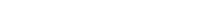# Math riddles Level 26 Answer with Hints and solutionsMath riddles game level 26 Answer with solution android game developed by Black games. Scroll Below to find details.

Math Riddles tests your IQ with mathematical puzzles. Challenge yourself with different levels of math puzzles and stretch the limits of your intelligence. Every IQ game is prepared with an approach of an IQ test. Improve your mathematics, challenge your brain with this game. There are many hard levels which you may not have solved then you can take help from here.

Math app level 26: 5 9 5 11 7 1 12 ?Solution: Sum of two adjacent numbers

there is any Doubt/discrepancy then please let me know in comments or you are unable to understand solution then I will surely help you.

### 5 thoughts on “Math Riddles App Level 26 Solution Android”

•November 14, 2019 at 3:00 am

You only add the numbers on the sloping sides …

4 + 7 = 11
7 + 12 = 19
5 + 4 = 9

12 + 5 = 17

•November 8, 2019 at 10:31 am

I still dont get how and why 17 is the answer… Elaborate pls..

•September 30, 2019 at 8:59 am

•May 17, 2019 at 1:27 am
•